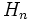# Normal sub-APS

Jump to: navigation, search

## Contents

BEWARE! This term is nonstandard and is being used locally within the wiki. [SHOW MORE]
This term is related to: APS theory
View other terms related to APS theory | View facts related to APS theory
ANALOGY: This is an analogue in APS of a property encountered in group. Specifically, it is a sub-APS property analogous to the subgroup property: normal subgroup
View other analogues of normal subgroup | View other analogues in APSs of subgroup properties (OR, View as a tabulated list)

## Definition

Let$(G,\Phi)$ be an APS of groups and$H$ be a sub-APS of$G$. We say that$H$ is normal in$G$ if the following equivalent conditions hold:

• For every$n$,$H_n$ is a normal subgroup of$G_n$
• There exists an APS of groups$(K,\Psi)$ and an APS homomorphism from$(G,\Phi)$ to$(K,\Psi)$ such that the kernel of the map at each$n$ is$H_n$